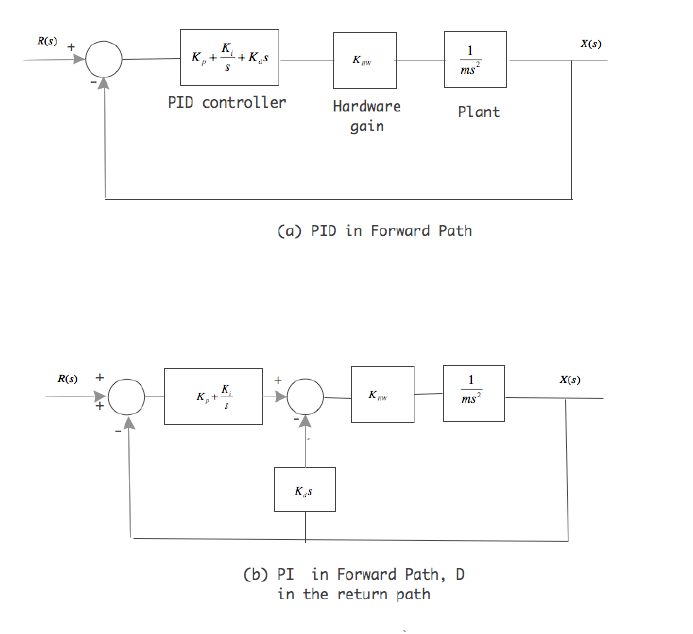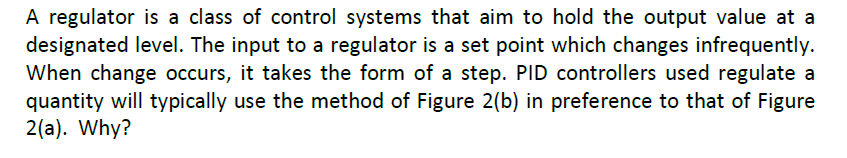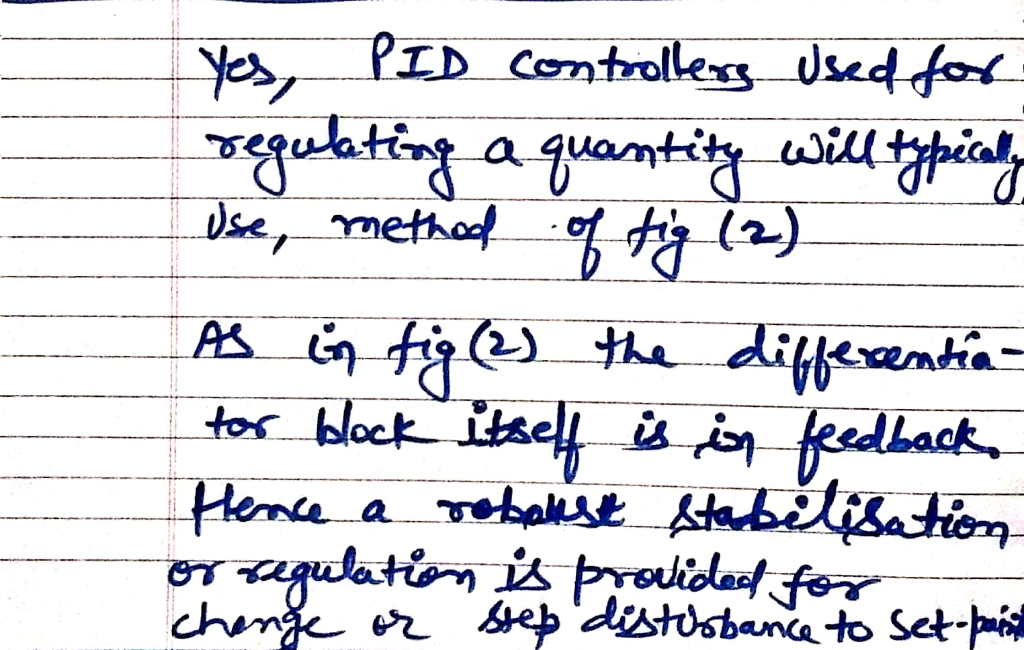Homework Help Question & Answers

# X(s) R(s) ms Hardware gain PID controller Plant (a) PID in Forward Path X(s) R(s)+ ms Kas (b) PI ...X(s) R(s) ms Hardware gain PID controller Plant (a) PID in Forward Path X(s) R(s)+ ms Kas (b) PI in Forward Path, D in the return path
A regulator is a class of control systems that aim to hold the output value at a designated level. The input to a regulator is a set point which changes infrequently. When change occurs, it takes the form of a step. PID controllers used regulate a quantity will typically use the method of Figure 2(b) in preference to that of Figure 2(a). Why?##### Add Answer of: X(s) R(s) ms Hardware gain PID controller Plant (a) PID in Forward Path X(s) R(s)+ ms Kas (b) PI ...
More Homework Help Questions Additional questions in this topic.

• #### System dynamics and control course. Use only “MATLAB “to solve this. Need a pro to help Let a system have plant transfer function (00.2) s3 +22s 156s+232 Design a PID controller such that the cl...

Need Online Homework Help?

Get FREE EXPERT Answers
WITHIN MINUTES
Related Questions